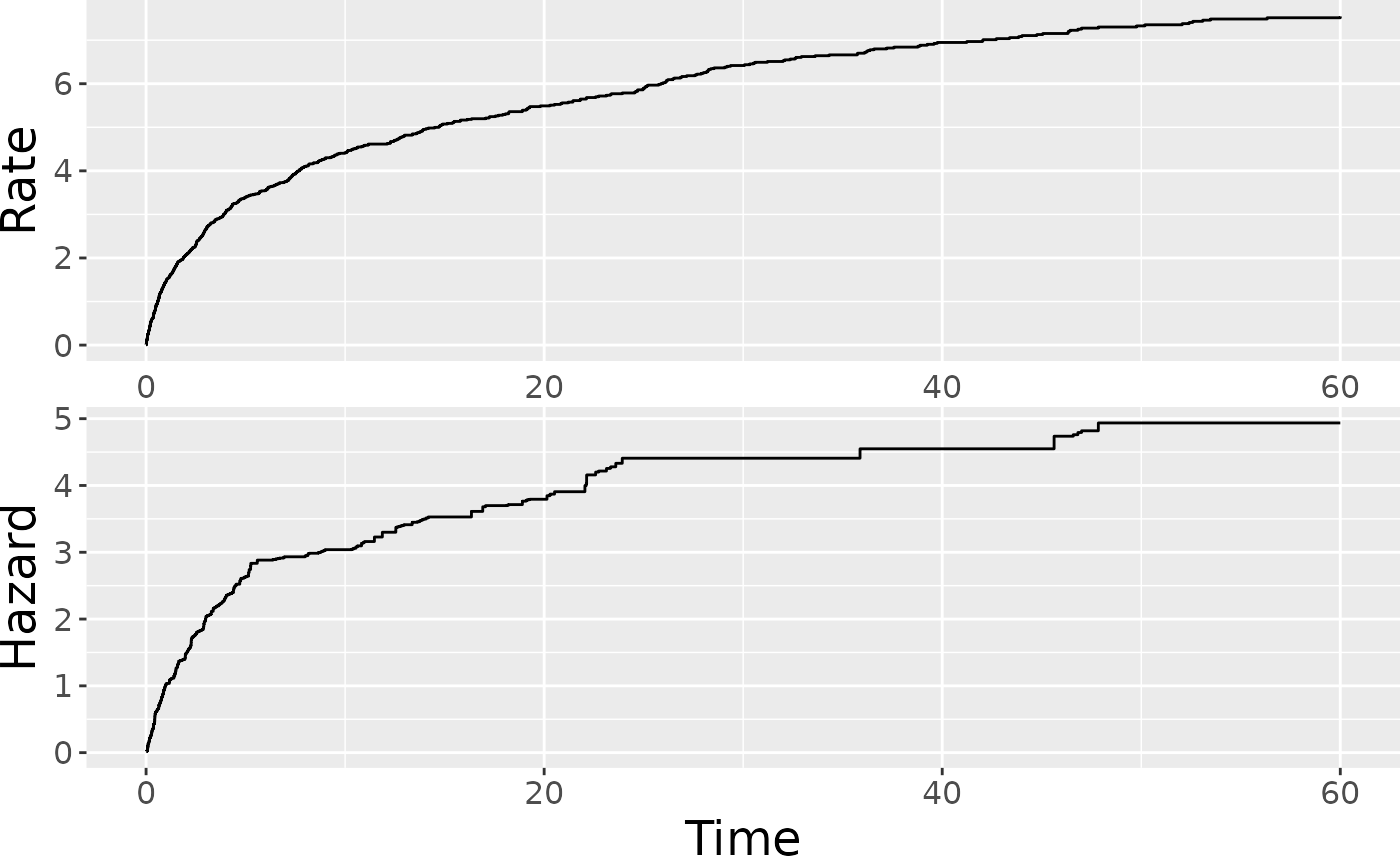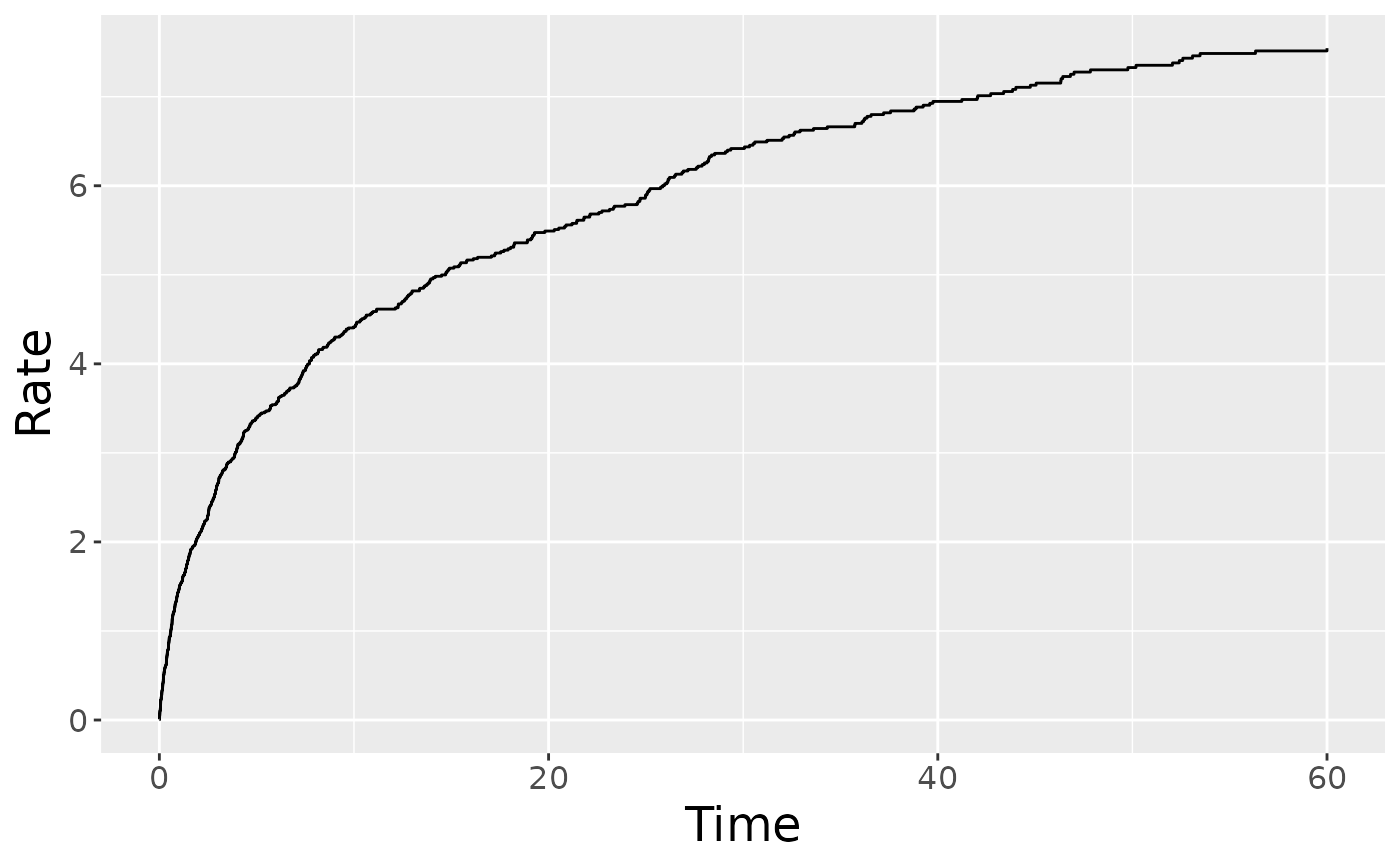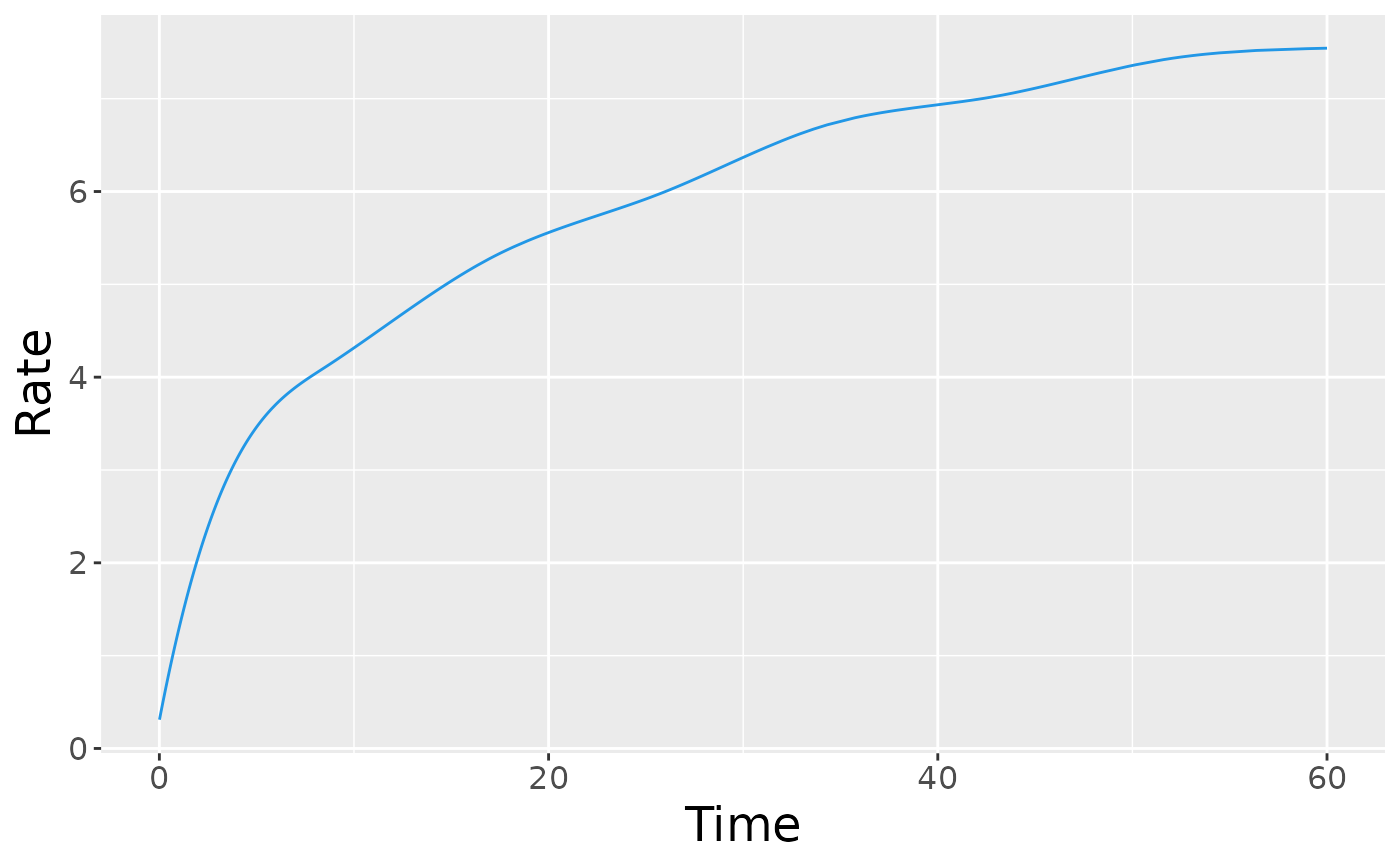Plot the baseline cumulative rate function for an reReg object.

plotRate(
x,
newdata = NULL,
frailty = NULL,
showName = FALSE,
type = c("unrestricted", "bounded", "scaled"),
smooth = FALSE,
control = list(),
...
)

## Arguments

x an object of class reReg, usually returned by the reReg function. an optional data frame contains variables to include in the calculation of the cumulative rate function. If omitted, the baseline rate function will be plotted. an optional vector to specify the shared frailty for newdata. If newdata is given and frailty is not specified, the an optional logical value indicating whether to label the curves when newdata is specified. a character string specifying the type of rate function to be plotted. Options are "unrestricted", "scaled", "bounded". See Details. an optional logical value indicating whether to add a smooth curve obtained from a monotone increasing P-splines implemented in package scam. a list of control parameters. graphical parameters to be passed to methods. These include xlab, ylab, main, and more. See Details.

## Value

A ggplot object.

## Details

The plotRate() plots the estimated baseline cumulative rate function depending on the identifiability assumption. When type = "unrestricted" (default), the baseline cumulative rate function is plotted under the assumption $$E(Z) = 1$$. When type = "scaled", the baseline cumulative rate function is plotted under the assumption $$\Lambda(\min(Y^\ast, \tau)) = 1$$. When type = "bounded", the baseline cumulative rate function is plotted under the assumption $$\Lambda(\tau) = 1$$. See ?reReg for the specification of the notations and underlying models.

The argument control consists of options with argument defaults to a list with the following values:

xlab

customizable x-label, default value is "Time".

ylab

customizable y-label, default value is empty.

main

customizable title, default value is "Baseline cumulative rate function".

These arguments can also be specified outside of the control list.

reReg plot.reReg

## Examples

data(simDat)
fm <- Recur(t.start %to% t.stop, id, event, status) ~ x1 + x2
fit <- reReg(fm, data = simDat, model = "cox|cox", B = 0)
## Plot both the baseline cumulative rate and hazard function
plot(fit)## Plot baseline cumulative rate function
plotRate(fit)plotRate(fit, smooth = TRUE)